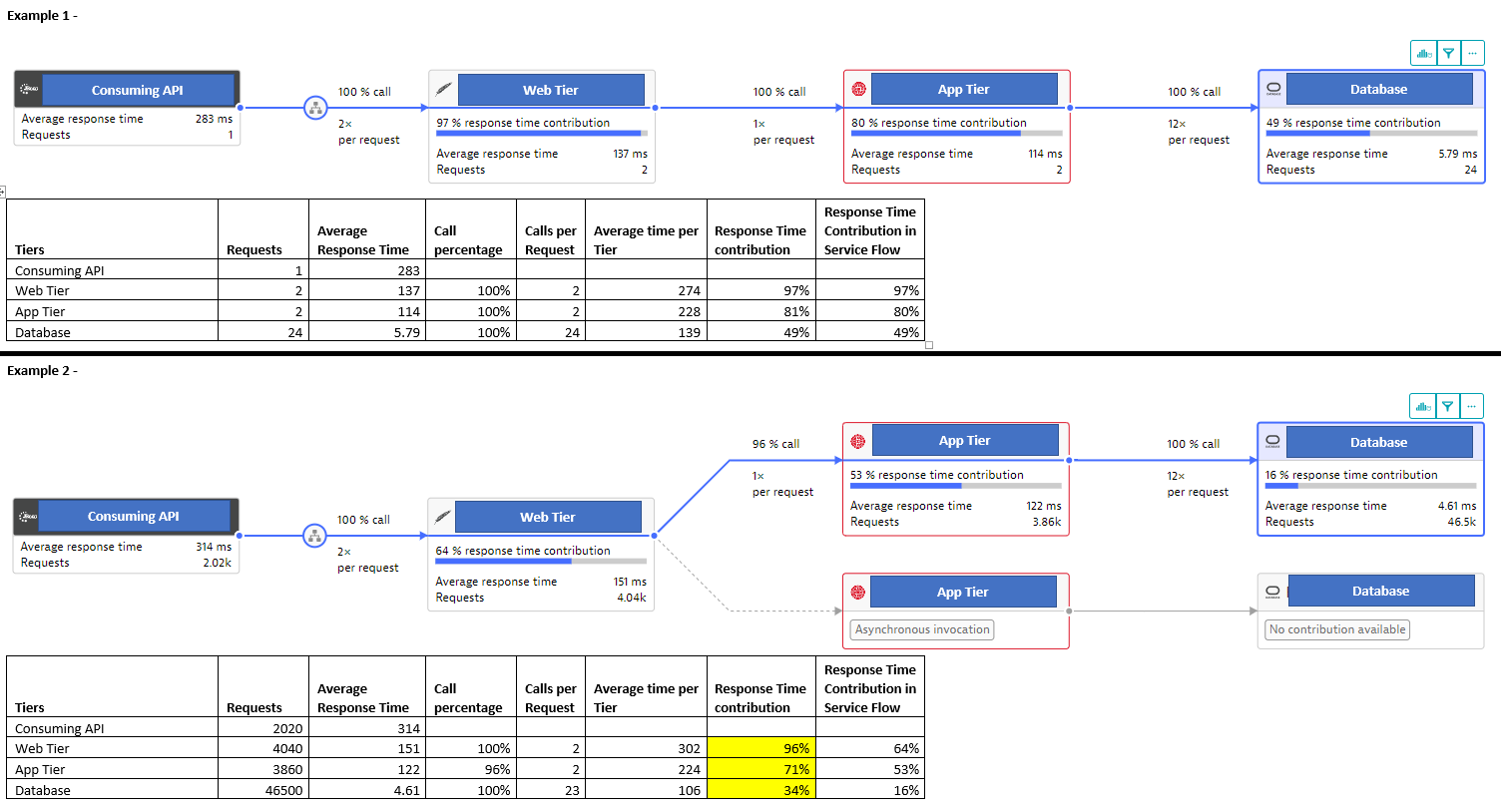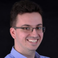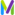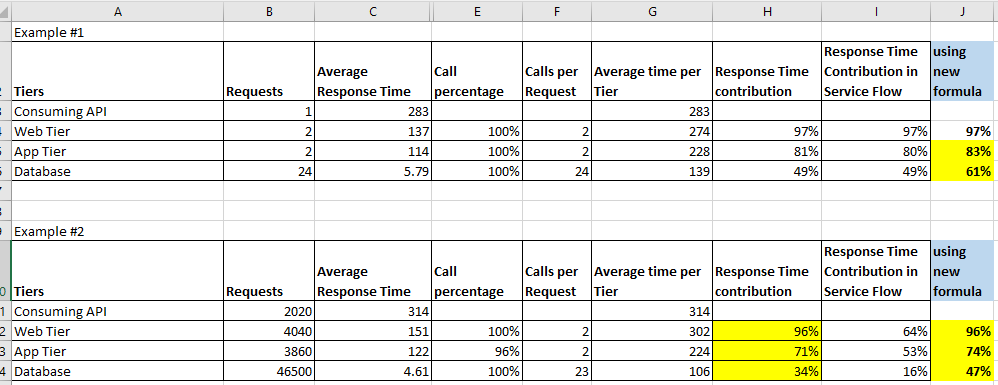cancel
Showing results for
Show  only  | Search instead for
Did you mean:

## How is the response time contributions calculated in complex service flows?Newcomer

I have been trying to explain to a project how response time contribution is calculated in a Service Flow diagram. I have been referring to this answer but came across an inconsistency in the calculation results for more complex service flows. Can someone please tell me how response time contributions are calculated and why my calculations seem to be off in Example #2 attached?Excel calculations examples-calcs.xlsx

In Example 1 –

• In
this case I am using the service flow for a single PurePath
• The
calculations in this case are mostly adding up – The response time
contribution calculation matches the contribution reported in the service
flow

In Example 2 –

• For
this case I use a service flow analyzing approx. 2k transactions.
• Using
the same formulas from example 1, the response time contributions no longer
match what we're seeing in the Service Flow

• There's
some asynchronous invocations in play here. But even the contribution
calculation for the Web Tier, which is upstream to the async calls, does
not match what is reported in the service flow

The formulas I'm
using:

• (Average
time per Tier) = (Average
Response Time) x (Call Percentage) x (Calls per request)
• (Calls per request) = (Requests) / (Consuming API.Requests)
• (Response Time Contribution) = (Average time per Tier) /
(Consuming API.Average Response Time)

Note: The first formula I got from this answer: https://community.dynatrace.com/t5/Dashboarding/How-is-the-response-time-contribution-in-service-flo...

2 REPLIES 2DynaMight Guru

I understand this like this

We have something like this:

Root —- A(60%) —- B (70%). —- C (20%)

This means that on A we spent 60%, from this time 70% was spent on component B (from root it will be x*0.6*0.7)

From this time 20% was spent on component C so x*0.6*0.7*0.2

Sebastian

Regards, Sebastian